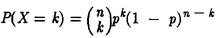# Section 13.6: Binomial Distribution

## 13.6 Outline

1. Random variables
1. definition
2. binomial random variable
3. binomial distribution
2. Binomial experiments
1. definition; four conditions
1. fixed number of trials
2. two mutually exclusive outcomes
3. trials are independent
4. probabilities remain constant for each trial
2. binomial distribution theorem
3. Bernoulli trials

## 13.6 Essential Ideas

Binomial Experiment

A binomial experiment is an experiment that meets four conditions.

1. There must be a fixed number of trials; denote this by n.
2. There must be two possible mutually exclusive outcomes for each trial. Call themsuccess and failure.
3. Each trial must be independent.
4. The probability of success and failure must remain constant for each trial.

Binomial Distribution Theorem

Let X be a random variable for the number of success in n independent and identical repetitions of an experiment with two possible outcomes, success and failure. If p is the probability of success, then for k = 0, 1, … , n,